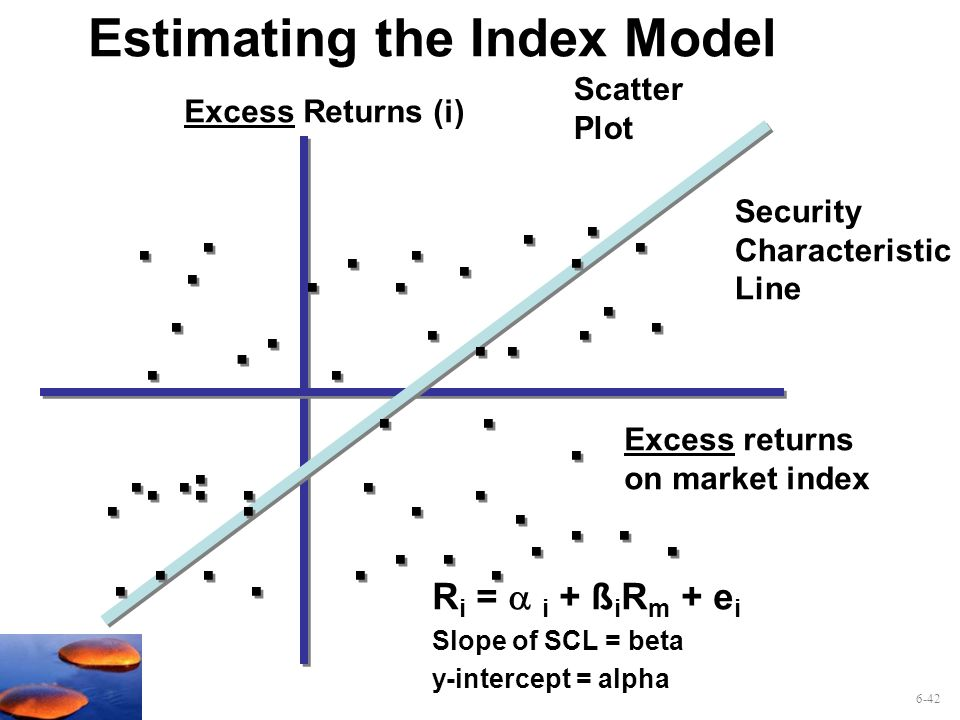# Reading 44 : Portfolio Risk and Return (P.2)

4. Explain return generating models (including the market model) and their uses

• Return generating models are used to estimate the expected returns on risky securities based on specific factors. A simplified ⇒ The market model:

Ri = ?i + ?i . Rm + ei

Where:       i: Return on asset i, Rm : market return.

?i: intercept, ?i =  (1-?i ) x Rf

?i : slope coefficient, ei: abnormal return on asset i.

• Multifactor Models: most commonly used macroeconomic, fundamental, statistic factors.
• Macroeconomic factors: GDP growth, inflation, consumer confidence…
• Fundamental factors: earnings, earnings growth, firm size & research expenditures…
• Statistic factors: have no basis in finance theory & specific time period data set.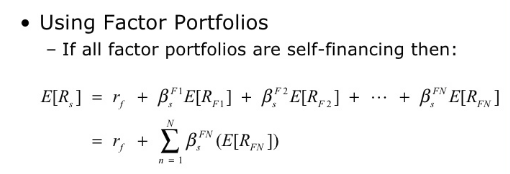The simplest factor is single-factor model (single-index model)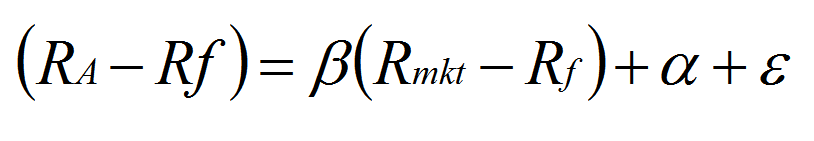5. Calculate and interpret beta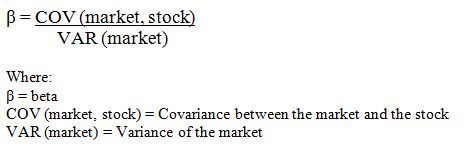Correlation between the returns on asset i with the return on the market index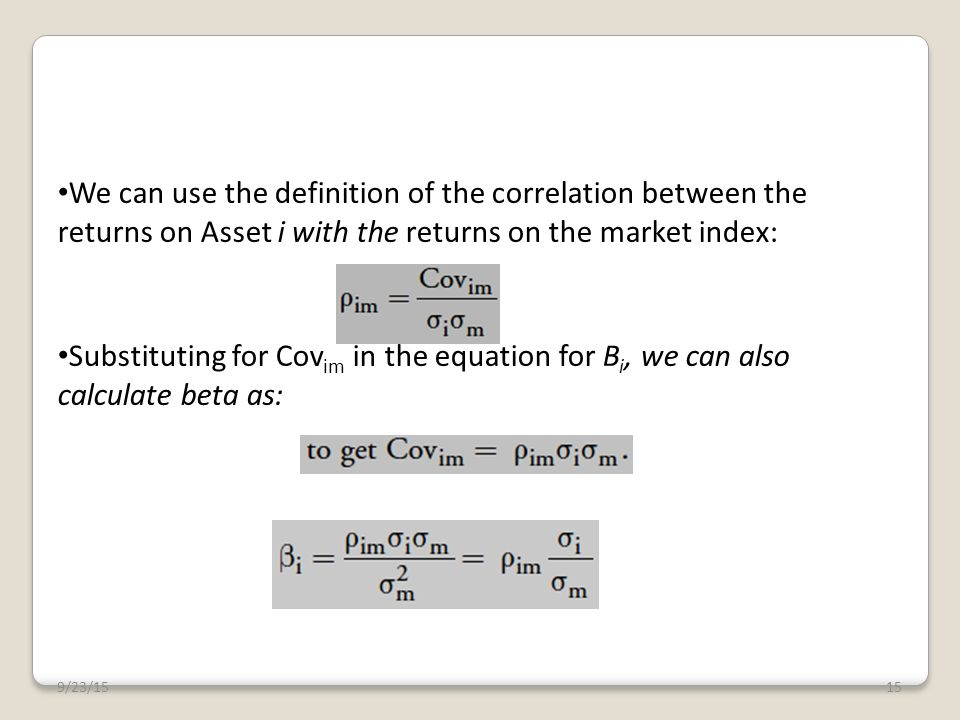• This regression line is referred to as the asset’s security characteristic line (SCL)